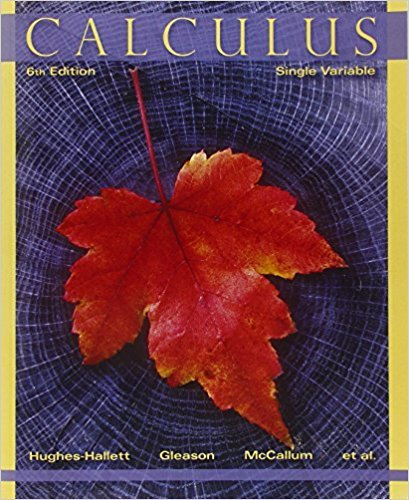×
×

# Solutions for Chapter 10.2: TAYLOR SERIES## Full solutions for Calculus: Single Variable | 6th Edition

ISBN: 9780470888643Solutions for Chapter 10.2: TAYLOR SERIES

Solutions for Chapter 10.2
4 5 0 272 Reviews
18
0
##### ISBN: 9780470888643

Chapter 10.2: TAYLOR SERIES includes 55 full step-by-step solutions. Since 55 problems in chapter 10.2: TAYLOR SERIES have been answered, more than 32414 students have viewed full step-by-step solutions from this chapter. This expansive textbook survival guide covers the following chapters and their solutions. Calculus: Single Variable was written by and is associated to the ISBN: 9780470888643. This textbook survival guide was created for the textbook: Calculus: Single Variable , edition: 6.

Key Calculus Terms and definitions covered in this textbook
• Complex fraction

See Compound fraction.

• Compounded k times per year

Interest compounded using the formula A = Pa1 + rkbkt where k = 1 is compounded annually, k = 4 is compounded quarterly k = 12 is compounded monthly, etc.

• equation of an ellipse

(x - h2) a2 + (y - k)2 b2 = 1 or (y - k)2 a2 + (x - h)2 b2 = 1

• Grapher or graphing utility

Graphing calculator or a computer with graphing software.

• Identity properties

a + 0 = a, a ? 1 = a

• Law of sines

sin A a = sin B b = sin C c

• Local extremum

A local maximum or a local minimum

• Lower bound of f

Any number b for which b < ƒ(x) for all x in the domain of ƒ

• Parameter interval

See Parametric equations.

• Polar distance formula

The distance between the points with polar coordinates (r1, ?1 ) and (r2, ?2 ) = 2r 12 + r 22 - 2r1r2 cos 1?1 - ?22

• Position vector of the point (a, b)

The vector <a,b>.

An angle in standard position whose terminal side lies on an axis.

• Reflexive property of equality

a = a

• Removable discontinuity at x = a

lim x:a- ƒ(x) = limx:a+ ƒ(x) but either the common limit is not equal ƒ(a) to ƒ(a) or is not defined

• Rigid transformation

A transformation that leaves the basic shape of a graph unchanged.

• Solve an equation or inequality

To find all solutions of the equation or inequality

A graph in which (-x, y) is on the graph whenever (x, y) is; or a graph in which (-r, -?) or (r, ?, -?) is on the graph whenever (r, ?) is

• Symmetric difference quotient of ƒ at a

ƒ(x + h) - ƒ(x - h) 2h

• Symmetric property of equality

If a = b, then b = a

• Variable

A letter that represents an unspecified number.

×